8.3 meters long ladder is leaning against the wall of the well, and its lower end is 1.2 meters from this wall.

How high from the bottom of a well is the top edge of the ladder?

x =  8.21 m

### Step-by-step explanation:

Try calculation via our triangle calculator.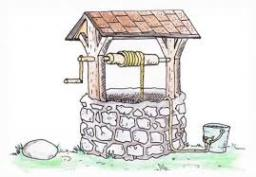Did you find an error or inaccuracy? Feel free to write us. Thank you!Tips to related online calculators
Pythagorean theorem is the base for the right triangle calculator.

#### You need to know the following knowledge to solve this word math problem:

We encourage you to watch this tutorial video on this math problem:

## Related math problems and questions:Ladder 6.4 meters long is positioned in the well such that its lower end is distanced from the wall of the well 1.2 m. The upper part of the ladder is supported on the upper edge of the well. How high is the well?The ladder has a length 3.5 meters. He is leaning against the wall so that his bottom end is 2 meters away from the wall. Determine the height of the ladder.Ladder 10 meters long is staying against the wall so that its bottom edge is 6 meters away from the wall. What height reaches ladder?Ladder 8 m long is leaning against the wall. Its foot is 1 m away from the wall. At which height ladder touches the wall?How long is a ladder that touches on a wall 4 meters high, and its lower part is 3 meters away from the wall?Adam placed the ladder of the house, the upper end reaching to the window at the height of 3.6m, and the lower end standing on level ground and was distant from a wall of 1.5m. What is the length of the ladder?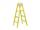The double ladder has 3 meters long shoulders. What is the height of the upper of the ladder reach if the lower ends are 1.8 meters apart?The double ladder is 8.5m long. It is built so that its lower ends are 3.5 meters apart. How high does the upper end of the ladder reach?The double ladder shoulders should be 3 meters long. What height will the upper top of the ladder reach if the lower ends are 1.8 meters apart?The ladder is 10 m long The ladder is 8 m high How many meters is the distant heel from the wall?The ladder has a length of 3 m and is leaning against the wall, and its inclination to the wall is 45°. How high does it reach?The ladder touch on a wall at a height of 7.5 m. The angle of the inclination of the ladder is 76°. How far is the lower end of the ladder from the wall?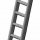Michael has a 35 foot ladder leaning against the side of his house. If the bottom of the ladder is 21 feet away from his house, how many feet above the ground does the ladder touch the house?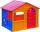How tall is the gable of a house in the shape of an isosceles triangle with a base length of 8 meters and an arm 5 meters long?Determine the volume of the concrete block whose one edge of the base has a length of 3 meters, body diagonal is 13 meters, and its height is 12 meters.4 m long ladder touches the cube 1mx1m at the wall. How high reach on the wall?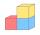Calculate the length of the wall diagonal of the cube with an edge 5 cm long.